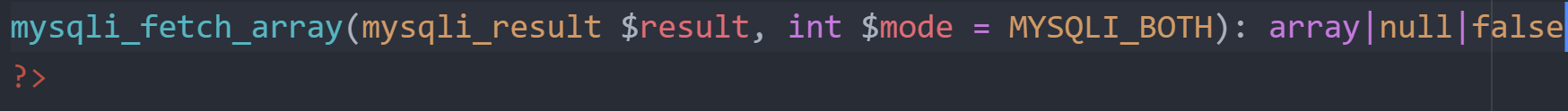PHP Tutorials
Aug 22, 2021

# Mysqli_Fetch_Array() Function Examples & Use Case - Grab Next Row

Here’s how we can use the mysqli_fetch_array() function in php to fetch the next row of data.Here’s how we can use the mysqli_fetch_array() function in php to fetch the next row of data.

# Mysqli_Fetch_Array() Function Introduction & Syntax

The Mysqli_fetch_array() function fetches one row of data from a result set and returns it as an associative or numeric array. This function has two different styles for the syntax for both object-oriented and procedural programming.

### Object-Oriented Style

``````
public mysqli_result::fetch_array(int \$mode = MYSQLI_BOTH)

: array|null|false
``````

### Procedural Style

``````
mysqli_fetch_array(mysqli_result \$result, int \$mode = MYSQLI_BOTH)

: array|null|false
``````

Each time this function is called on a given result set it will return the next row as an array or null if there are no more rows. The function stores the extracted data in the numeric indices of the returned output array but can also store the extracted data in associative indices by using the found field values as keys from the result set.

### Parameters For Mysqli_Fetch_Array():

These parameters differ based on procedural style vs object-oriented style.

#### Result

This function only exists in procedural style. It is a mysqli_result object passed by other mysqli functions.

#### Mode

This is an optional parameter that is used to pass a constant variable defining what type of array should be returned. The constant options are: MYSQLI_ASSOC, MYSQLI_NUM, OR MYSQLI_BOTH.

### Return Values For Mysqli_Fetch_Array() Function:

This function returns a php array made up of the data from the fetched row, or null if there are no more rows to read in from the result set variable, or false on a failure.

### Quick Notes About The PHP Mysqli_Fetch_Array() Function:

When using the MYSQLI_ASSOC constant for the \$mode parameter this function will act exactly as the mysqli_fetch_assoc() function. If you use the MYSQLI_NUM constant this function will act exactly as the mysqli_fetch_row() function.

Field names that are used as keys in associative arrays are case sensitive.

This function sets any null input fields to null again.

If multiple columns from the result have the same exact name the last column found is used and will overwrite the previous data. If you want to access multiple columns with the same exact name you should use the numeric version of the returned array.

This function was added in PHP version 5.

# PHP Mysqli_Fetch_Array() Function Examples:

Let’s look at a number of different ways we can use both the procedural style and object-oriented style.

## Procedural Style Example:

This code example walks through multiple steps before and after the actual mysqli_fetch_array() function call to give you a better understanding.

``````

if (mysqli_connect_errno()) {
echo "Failed to connect to MySQL: " . mysqli_connect_error();
exit();

}
``````

``````
\$sql = "SELECT Lastname, Hours FROM Employees ORDER BY Lastname";
\$result = mysqli_query(\$con,\$sql);
``````

Here we start with a simple mysql query to grab some data from a table. Notice we can use the mysqli_connect_errno() function to check for errors connecting.

``````
// Numeric array
\$row = mysqli_fetch_array(\$result, MYSQLI_NUM);
printf ("%s (%s)\n", \$row, \$row);
``````

Let’s run our fetch array function on the read in data with the numeric constant indicating to produce a numeric array.

``````
// Associative array
\$row = mysqli_fetch_array(\$result, MYSQLI_ASSOC);
printf ("%s (%s)\n", \$row["Lastname"], \$row["Age"]);
``````

We’re also going to run the same function with the same current row data but with the associative constant to compare result row arrays.

``````
mysqli_free_result(\$result);
mysqli_close(\$con);
``````

Finally we’ll free the data and close our connection! The results are exactly the same when printed, but we access the two different types of arrays differently.

## Object-Oriented Style Example

Let’s look at how the mysqli_fetch_array() function works when using object oriented programming for both numeric and associative arrays.

``````
mysqli_report(MYSQLI_REPORT_ERROR | MYSQLI_REPORT_STRICT);
\$mysqli = new mysqli("localhost", "my_user", "my_password", "users");

\$query = "SELECT Name, Hours FROM Users ORDER BY ID LIMIT 2";
\$result = \$mysqli->query(\$query);
```
```

We’ll start by querying the database table to grab some user data.

``````
\$row = \$result->fetch_array(MYSQLI_NUM);
printf("%s (%s)\n", \$row, \$row);
```
```

We can create a numeric array and print it out as we did before, but this time we call “->fetch_array” to create the array. The \$result row variable is a mysqli result object.

``````
\$row = \$result->fetch_array(MYSQLI_ASSOC);
printf("%s (%s)\n", \$row["Name"], \$row["Hours"]);
``````

Here’s the same functionality we see above but with an associative array that has keys we can directly call.

``````
/* associative and numeric array */
\$row = \$result->fetch_array(MYSQLI_BOTH);
printf("%s (%s)\n", \$row, \$row["Hours"]);
``````

Lastly we will pass the MYSQLI_BOTH constant to produce an array with both options available. You can see we can access variables through a numeric key and a string key. Remember if this function fails we return a php null value

## Numeric Array & Associative Array Example

``````
\$row = \$result->fetch_array(MYSQLI_BOTH);
printf("%s (%s)\n", \$row["FirstName"], \$row);
``````

This simply prints the values in this given array for these two keys.

## Use Case Example For Mysqli_Fetch_Array()

Given this function is mainly used for database related operations, many use cases that involve big data and big data training can see the myqli_fetch_array() function come in handy. This function is perfect for loading data up from a database into a user dashboard or website. We’ve used this function when working with sentiment analysis data to load a fetched row into a user dashboard for them to confirm these examples are valid. It’s a nice and quick way to get user feedback on NLP results which is always part of the process.As we’ve seen from the above examples we can fetch a result row with two or more columns from this dataset into a single array.

# Summary

We've seen how we can fetch a row of data and add it to a result array.

## Instant Access To PHP Case Studies

Read real use cases and whitepapers using PHP